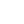# Javascript: How to Swap Two Elements in Array

By Henri ParviainenThis quick tutorial introduces two methods for swapping array elements using Javascript.

## Method 1 - Swap by using a temporary variable

We can swap array elements by declaring a temporary variable `temp` and placing the value in index `arr[x]` to it. Then, we can just set value in index `arr[y]` to `arr[x]` and finally, we can assign the value in `temp` to `arr[y]`. Voila, elements are now swapped.

``````const testArray = [1, 2, 3];

const swapElements = (arr, pos1, pos2) => {
const temp = arr[pos1];

arr[pos1] = arr[pos2];

arr[pos2] = temp;

return arr;
};

swapElements(testArray, 0, 2);

// [3, 2, 1];``````

## Method 2 - Single line solution

There is also a single-line solution we can use to swap elements in a Javascript array.

``````const testArray = [1, 2, 3];

const swapElements = (arr, x, y) => {
[arr[x], arr[y]] = [arr[y], arr[x]];

return arr;
};

swapElements(testArray, 0, 2);

// [3, 2, 1]``````

That is some clean-looking code and a good solution for swapping items in a Javascript array. However, in my opinion, it's not as easy to read as the first one. For that reason, I prefer to go with the first swap method, but the choice is yours!